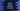# Python program to convert millimeters to inches## Python program to convert millimeters to inches:

In this post, we will learn how to convert a millimeter value to inches in Python. We will write one function that will take the millimeter value as input from the user and it will print the converted inch value.

## Formula to convert millimeter to inch:

To convert millimeter to inch, you need to divide it by 25.4. So,

``1 millimeter = 1/25.4 inch``

and

``x millimeter = (x/25.4) inch``

where x is any value in millimeter.

## Python program to convert millimeter to inch:

Let’s write down the python program to convert millimeter to inch:

``````milli = float(input("Enter the millimeter value: "))

inch = milli/25.4

print("Inches: ", inch)``````

In this program,

• We are reading the user input value using input. input returns the value in string. We are converting it to float. This is the millimeter value.
• The inch is calculated by dividing the millimeter by 25.4.
• The last line is printing the inch value.

If you run this program, it will print output as like below:

``````Enter the millimeter value: 122
Inches:  4.803149606299213``````

### Rounding the result:

We can round the result to a given number of digits after the decimal. Python provides one method called round, we can use that.

Let’s change the above program:

``````milli = float(input("Enter the millimeter value: "))

inch = round(milli/25.4, 2)

print("Inches: ", inch)``````

It will give output as like below:

``````Enter the millimeter value: 122
Inches:  4.8

Enter the millimeter value: 133
Inches:  5.24``````

## Python program to convert millimeter to inch by using a separate function:

We can use a separate function to do the conversion. If you are using millimeter to inch conversion in many places, creating a separate function makes it easier. You can call it from any other place of any other files. If you make any mistake, you need to change at one place.

Below program uses a separate function to do the millimeter to inch conversion:

``````def milliToInch(num):
return round(num/25.4, 2)

milli = float(input("Enter the millimeter value: "))

print("Inches: ", milliToInch(milli))``````

If you run this program, it will print similar output.